# 最大索引堆（IndexMaxHeap）

### 一、概念及其介绍

1. 优化了交换元素的消耗。
2. 加入的数据位置固定，方便寻找。

### 二、结构图示实现分析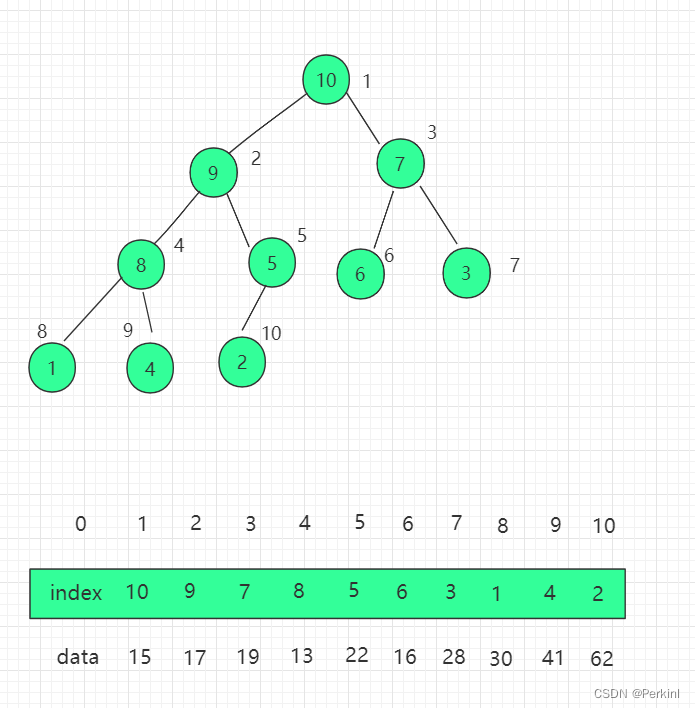``private T[] data;         // 最大索引堆中的数据private int[] indexes;    // 最大索引堆中的索引private int count;        // 索引堆中元素个数private int capacity;     // 表示堆的容量``

``public IndexMaxHeap(int capacity) {    data = (T[]) new Comparable[capacity + 1];    indexes = new int[capacity + 1];    count = 0;    this.capacity = capacity;}``

``public void insert(int i, T item) {    assert count + 1 <= capacity;    assert i + 1 >= 1 && i + 1 <= capacity;    i += 1;    data[i] = item;    // 调整插入操作，indexes 数组中添加的元素是真实 data 数组的索引 indexes [count+1] = i    indexes[count + 1] = i;     count++;    shiftUp(count);}``

``private void shiftUp(int k) {    while (k > 1 && data[indexes[k / 2]].compareTo(data[indexes[k]]) < 0) {        swapIndexes(k, k / 2); // 交换索引        k /= 2;    }}``

``public T extractMax() {    assert count > 0;    T ret = data[indexes];    swapIndexes(1, count);    count--;    shiftDown(1);    return ret;}``

``private void shiftDown(int k) {    while (2 * k <= count) {        int j = 2 * k;        if (j + 1 <= count && data[indexes[j + 1]].compareTo(data[indexes[j]]) > 0)            j++;        if (data[indexes[k]].compareTo(data[indexes[j]]) >= 0)            break;        swapIndexes(k, j);        k = j;    }}``

``public void change(int i, T newItem) {    i += 1;    data[i] = newItem;    // 找到indexes[j] = i, j表示data[i]在堆中的位置    // 之后shiftUp(j), 再shiftDown(j)    for (int j = 1; j <= count; j++)        if (indexes[j] == i) {            shiftUp(j);            shiftDown(j);            return;        }}``

### 三、完整代码示例

https://github.com/perkinls/java-summary/##### 热门推荐
•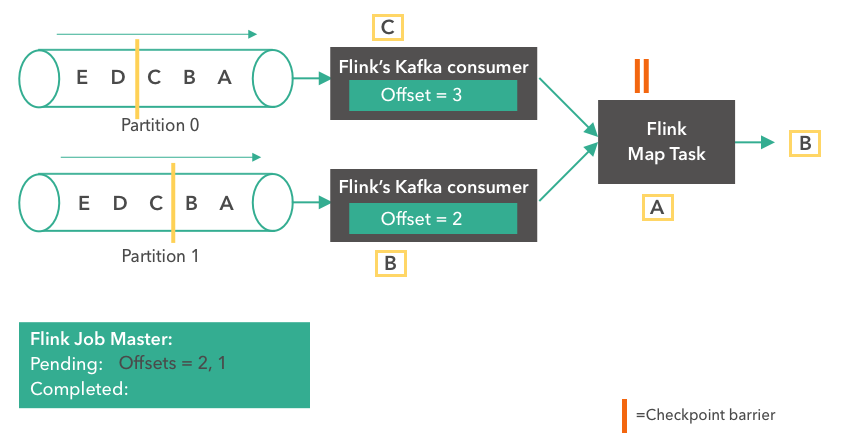浏览(2,548)
•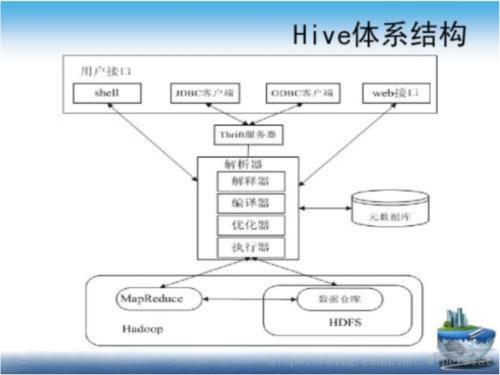浏览(1,776)
•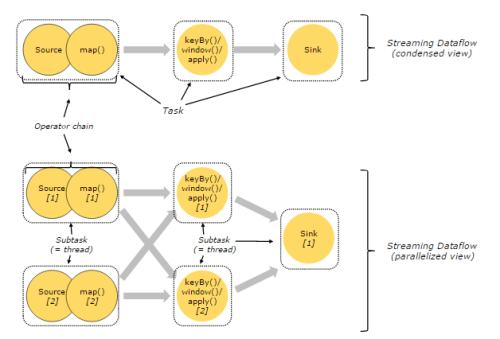浏览(1,739)
•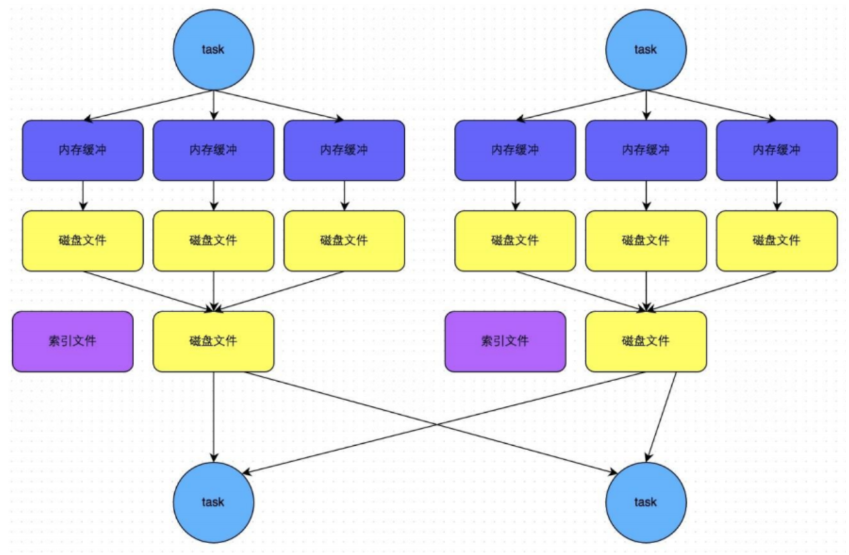浏览(1,732)
•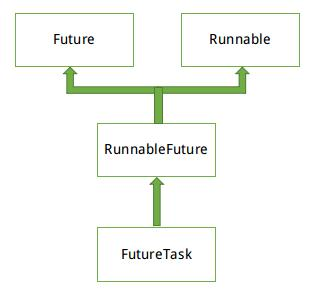浏览(1,685)
•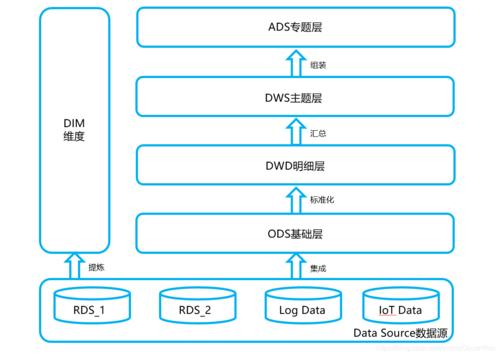浏览(1,673)
•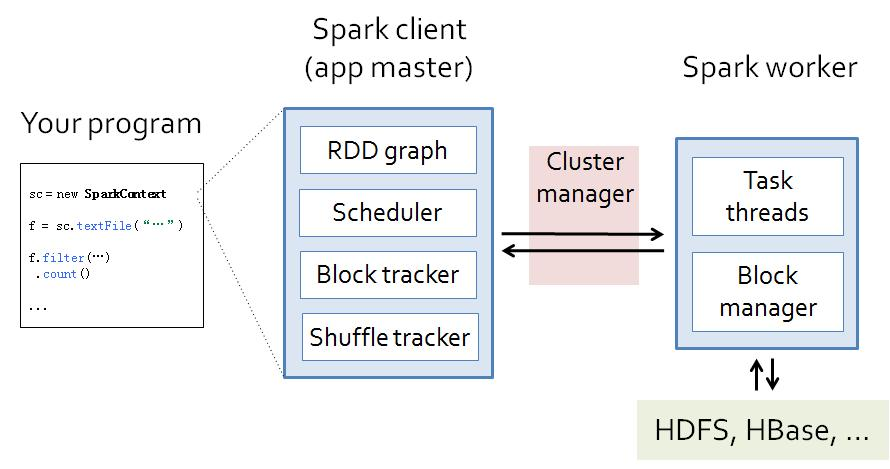浏览(1,640)
•浏览(1,613)
•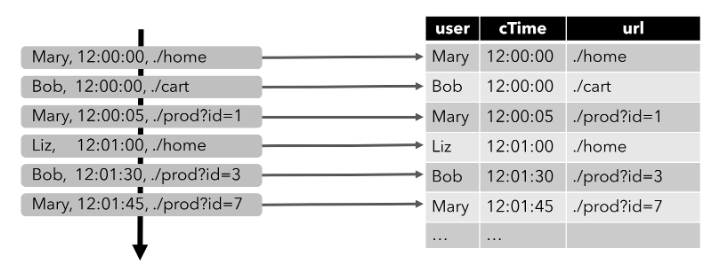浏览(1,537)
•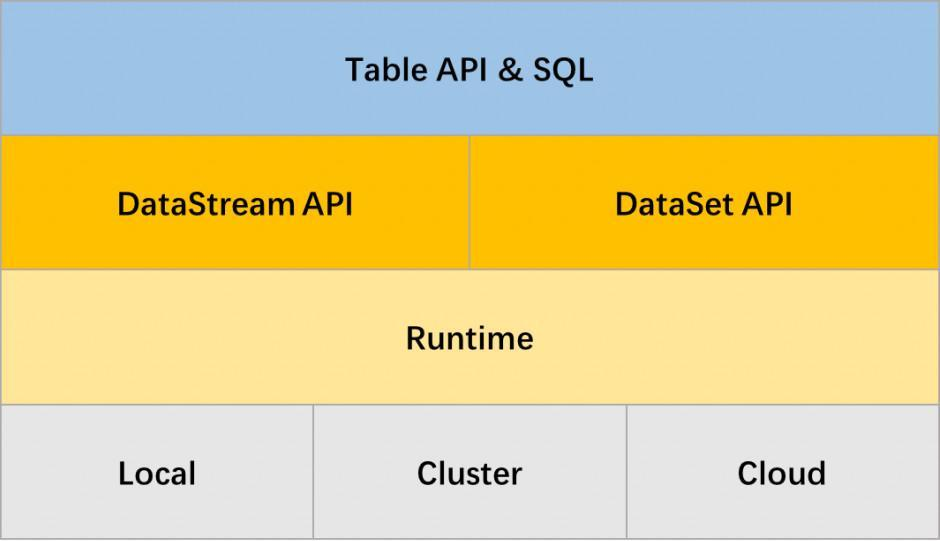浏览(1,474)